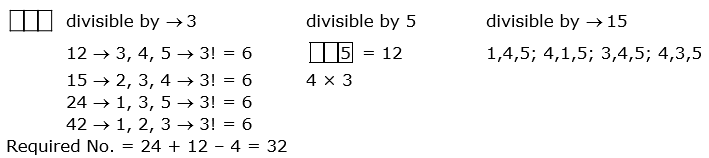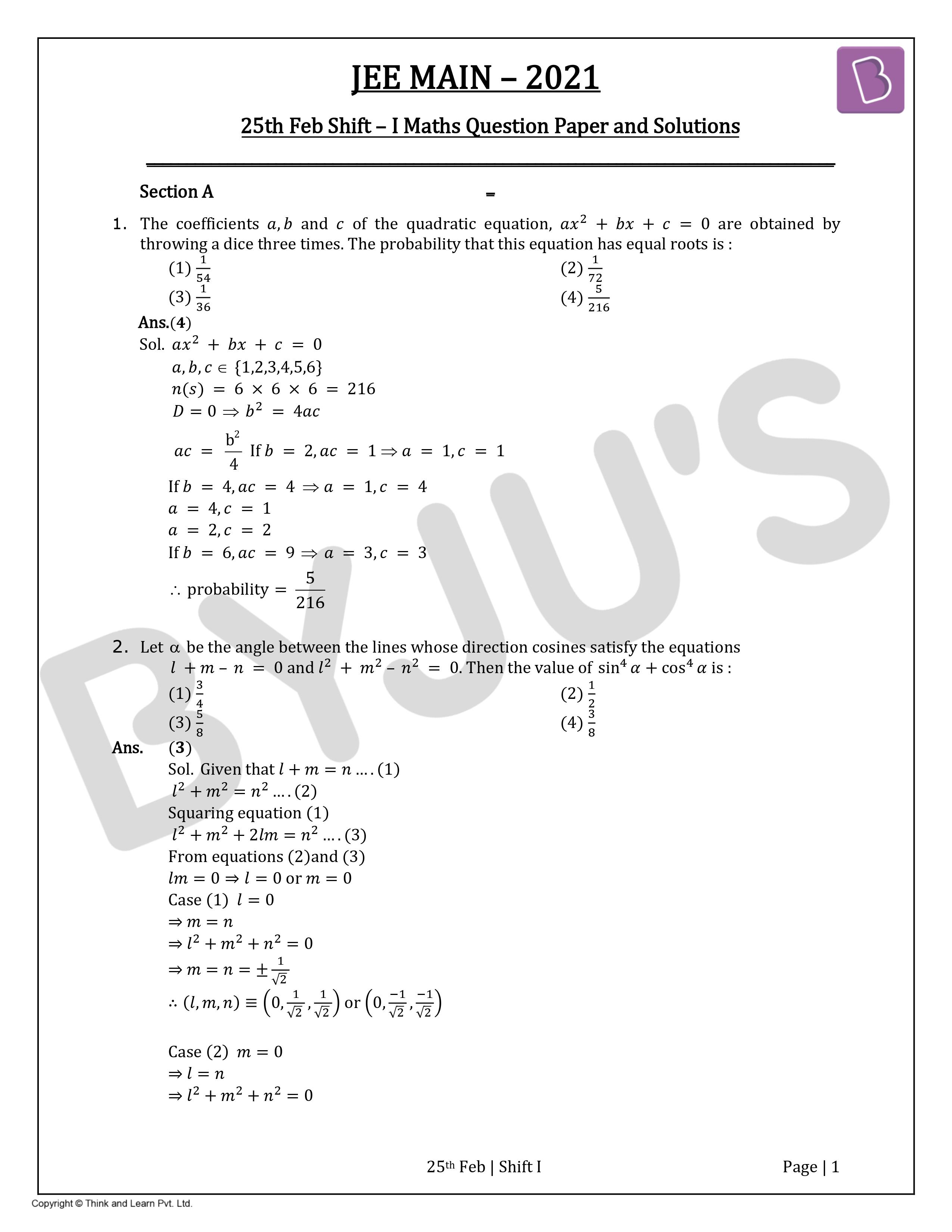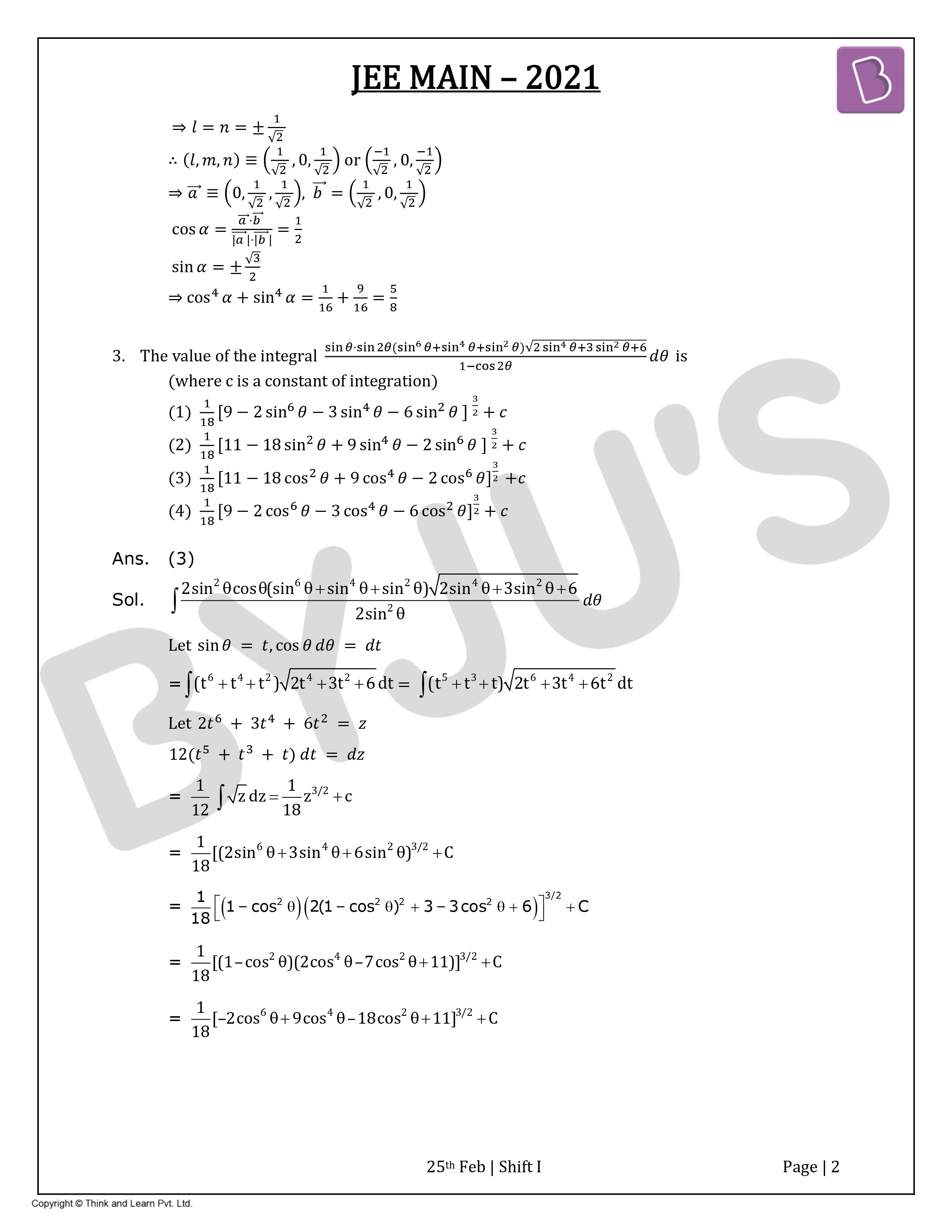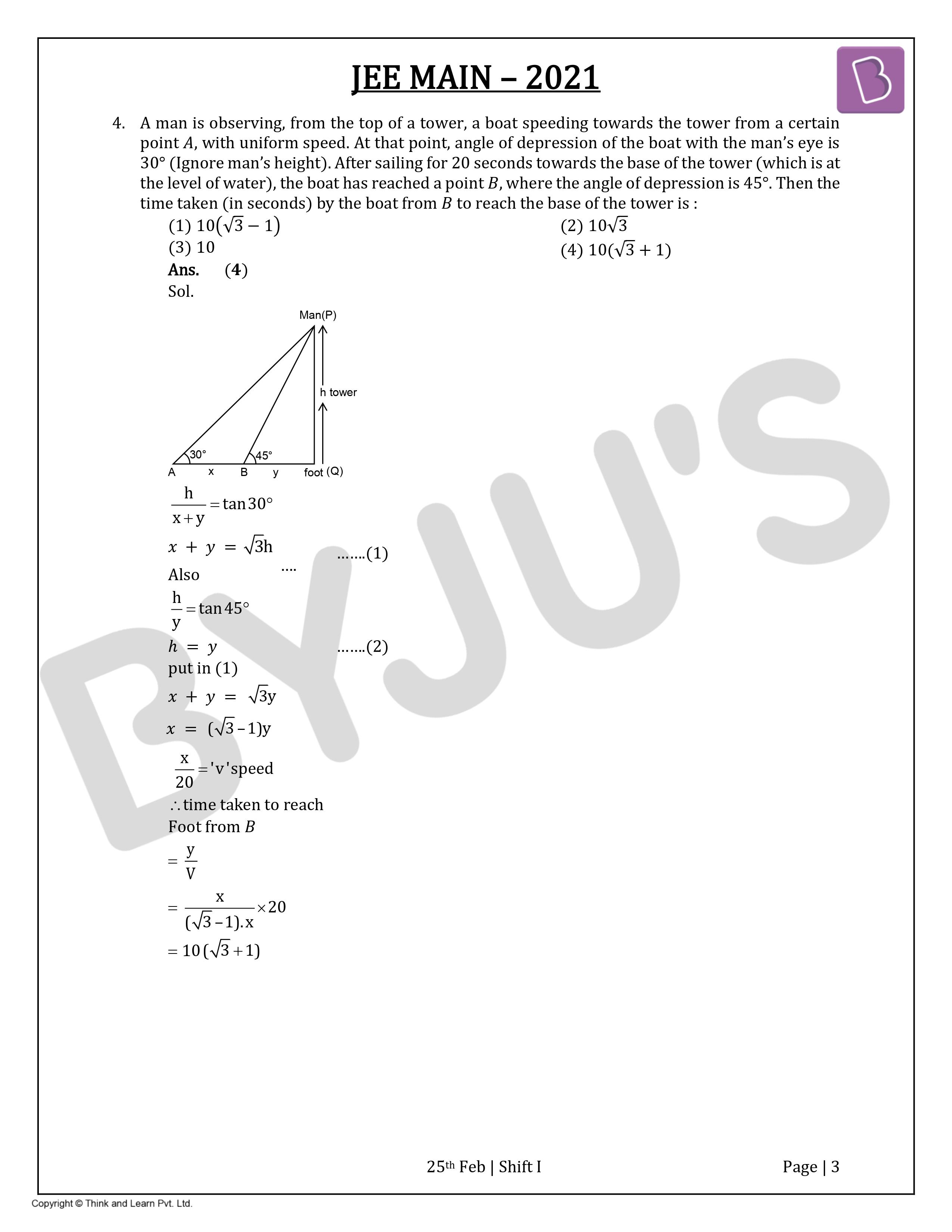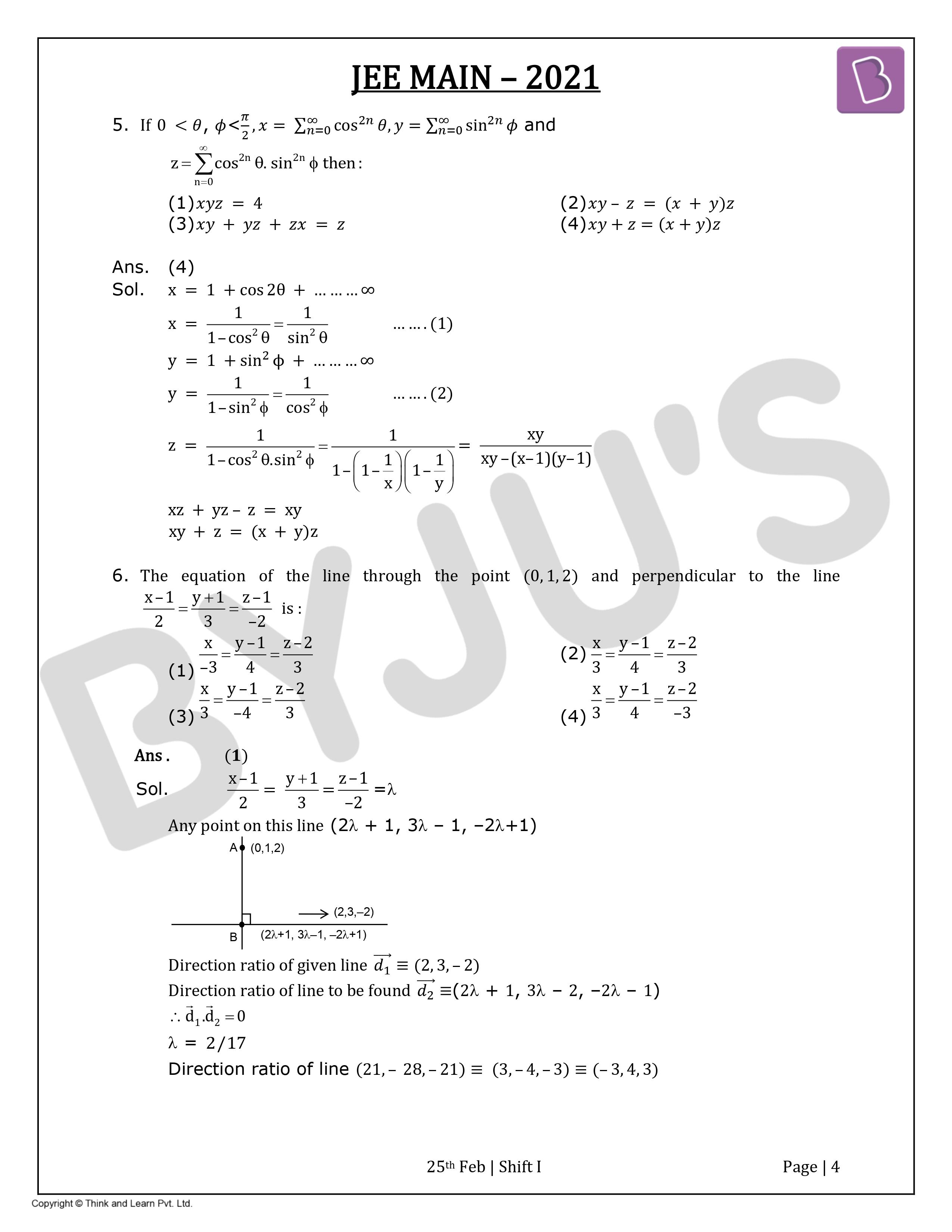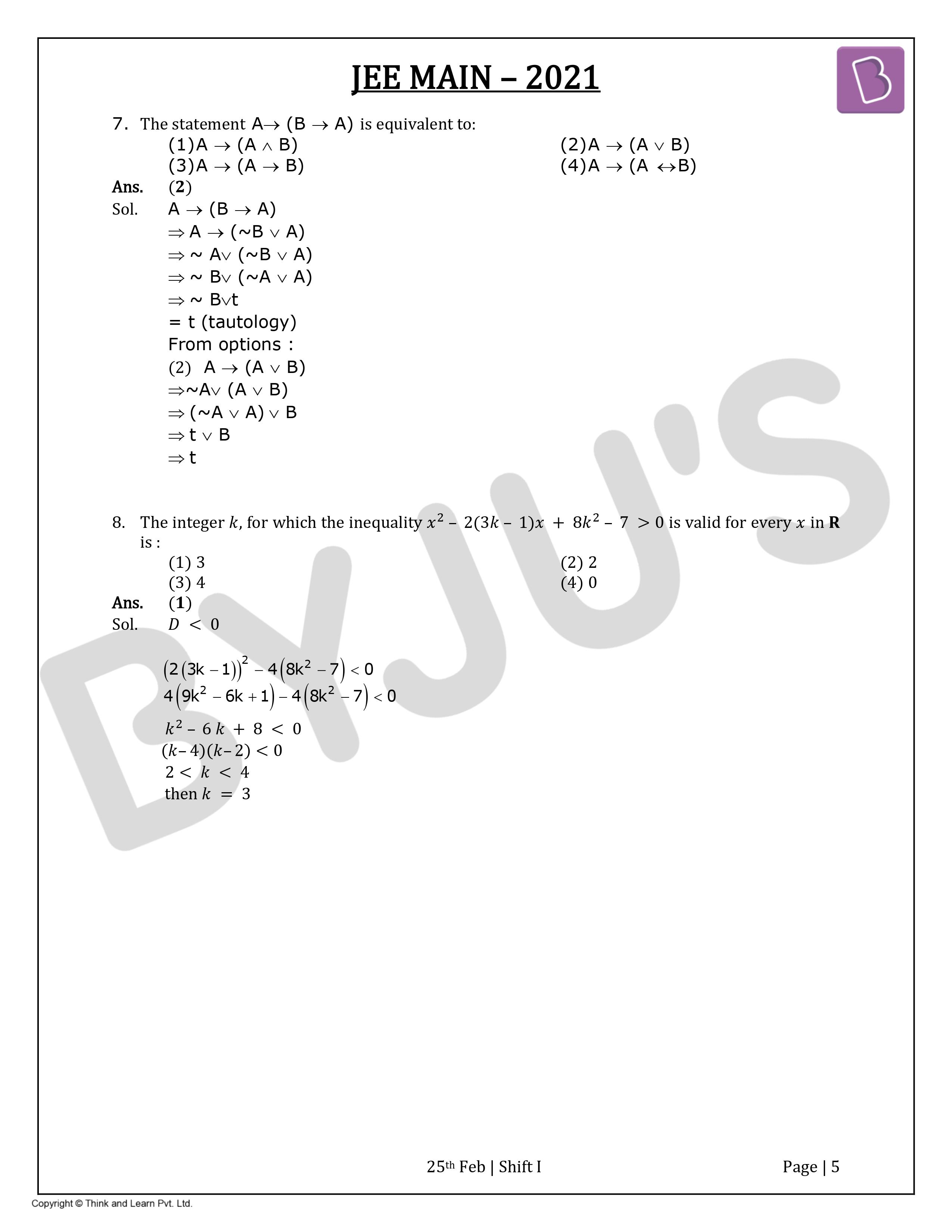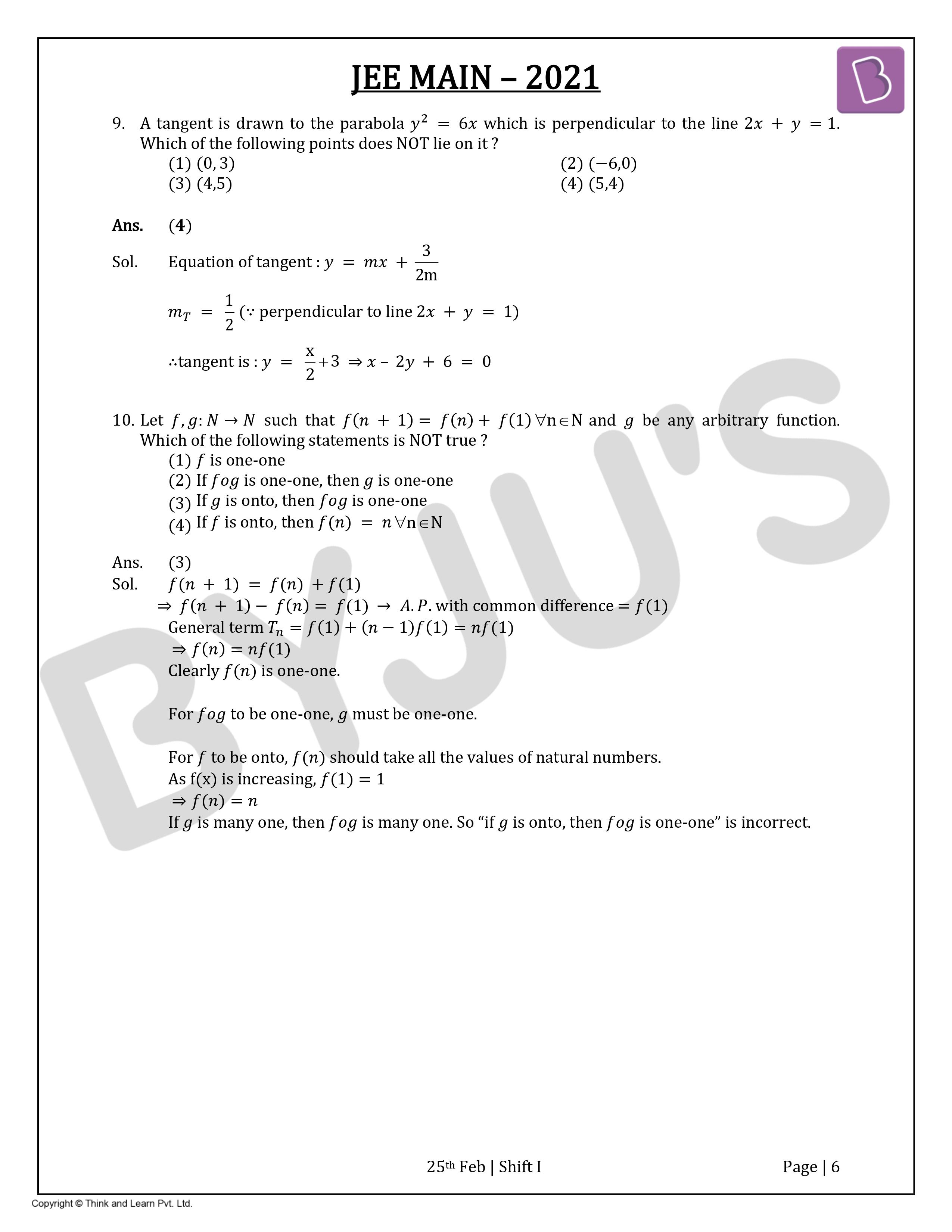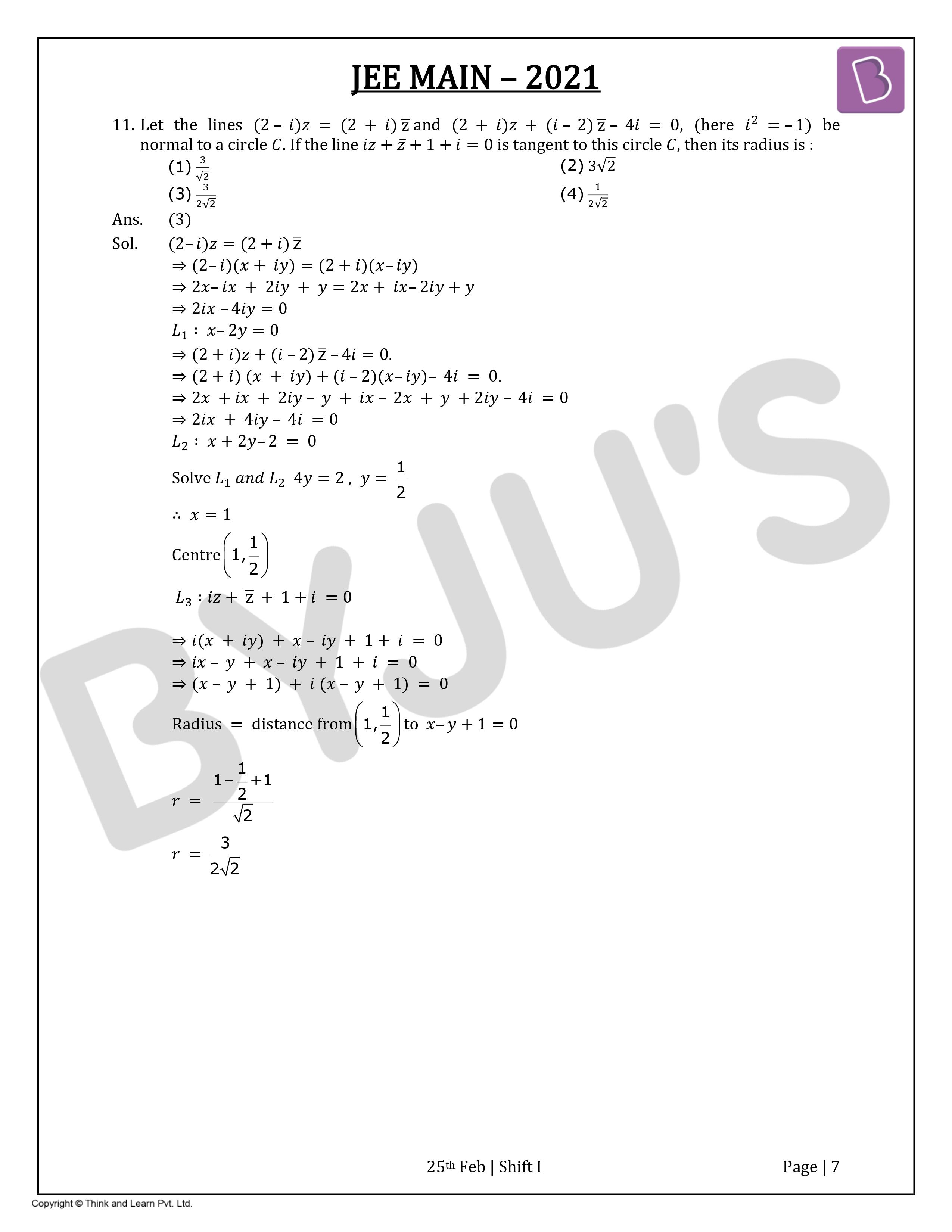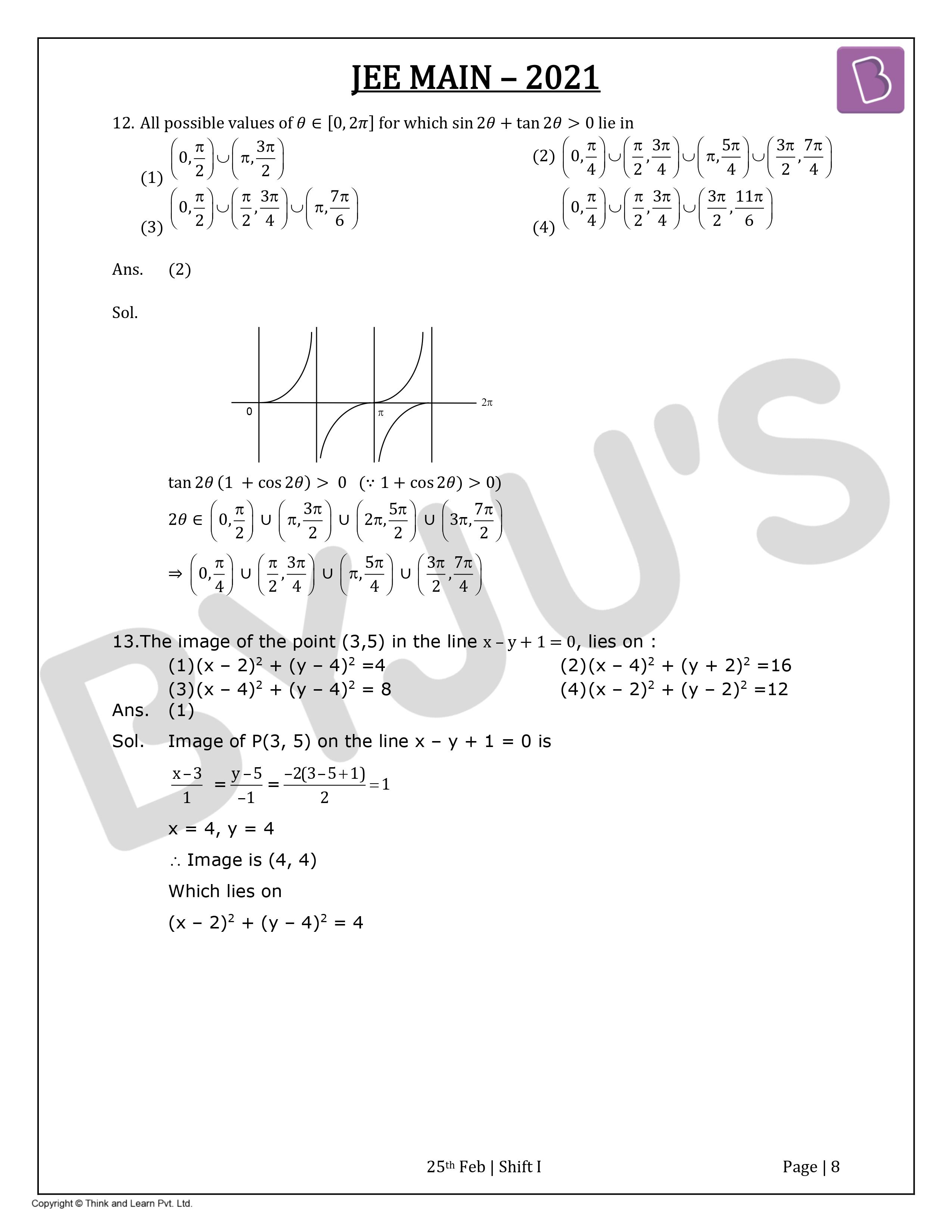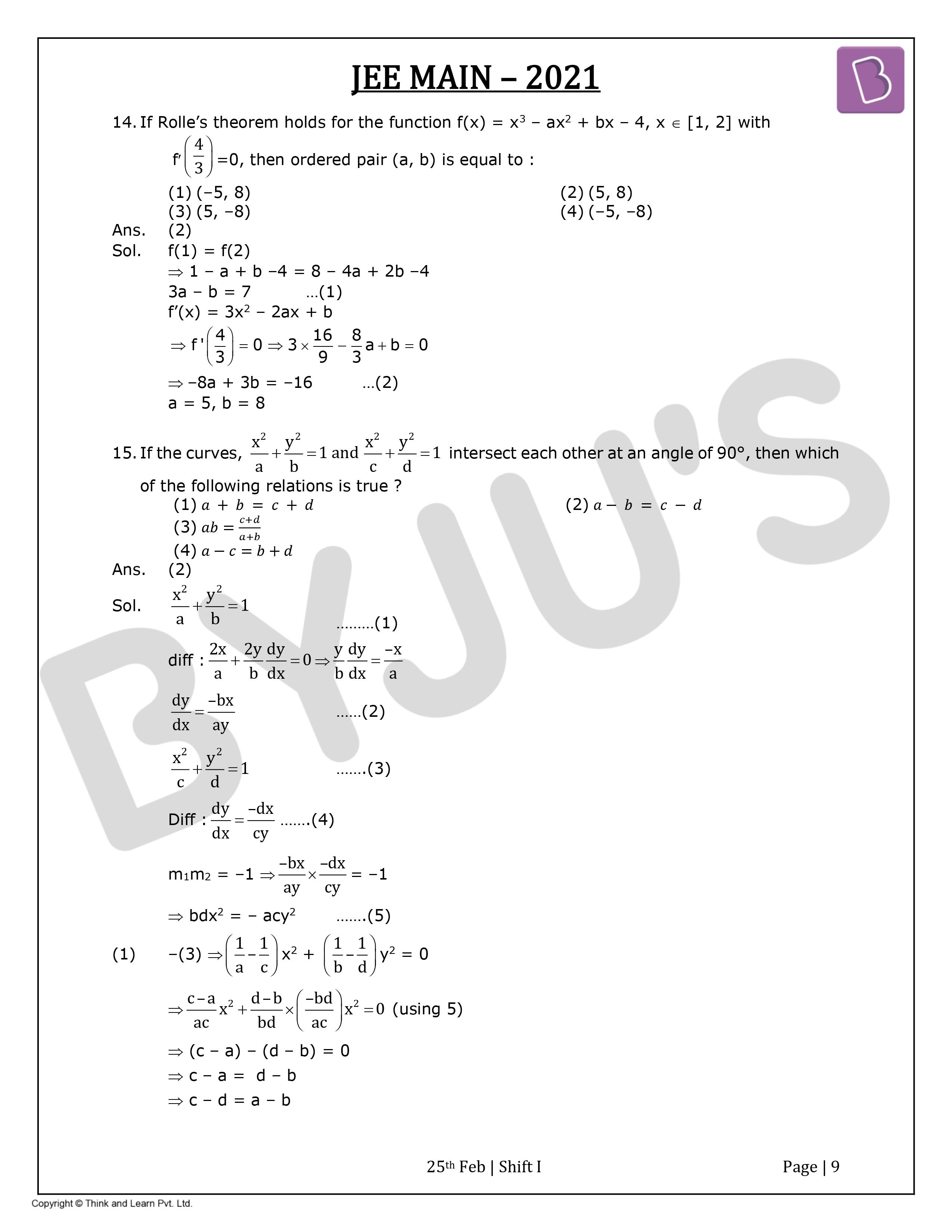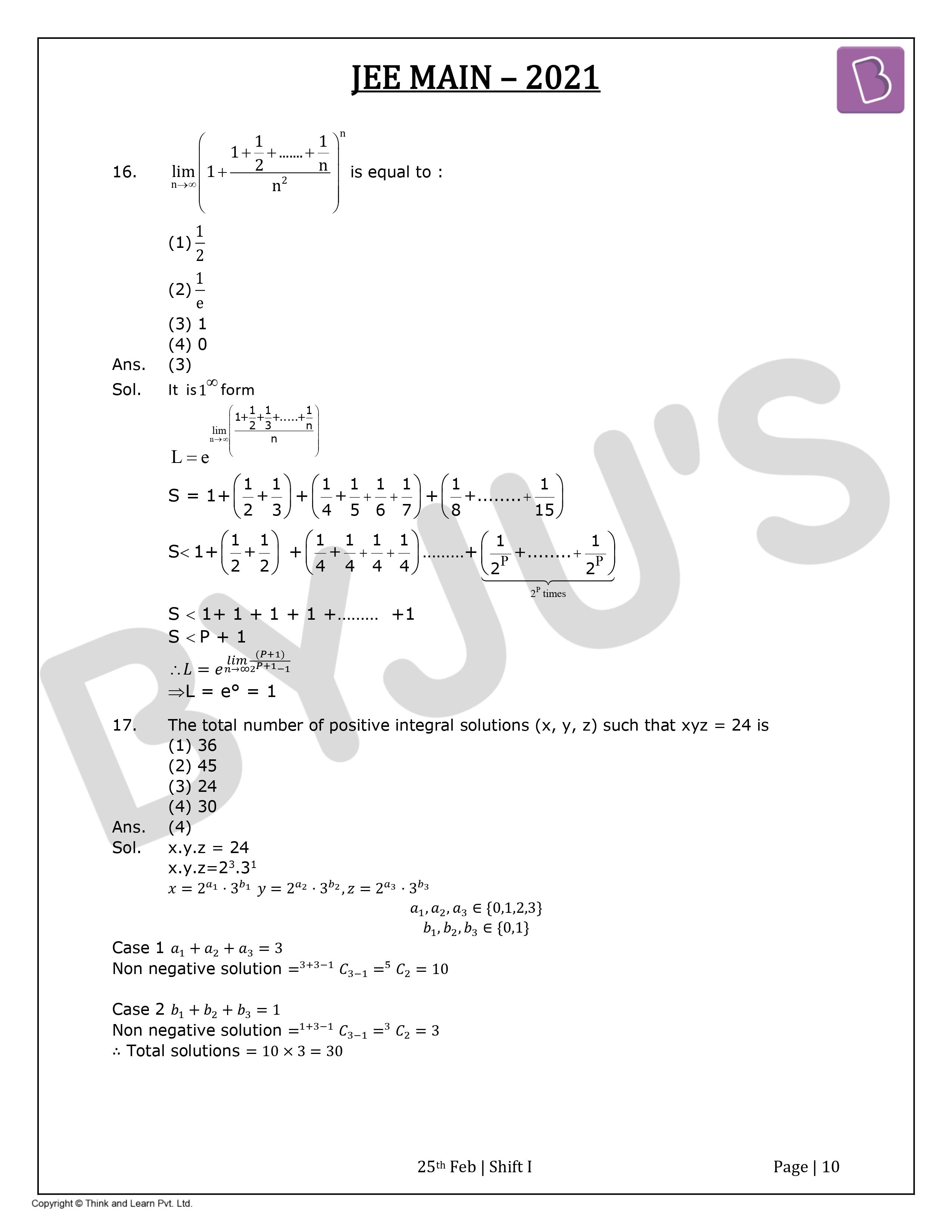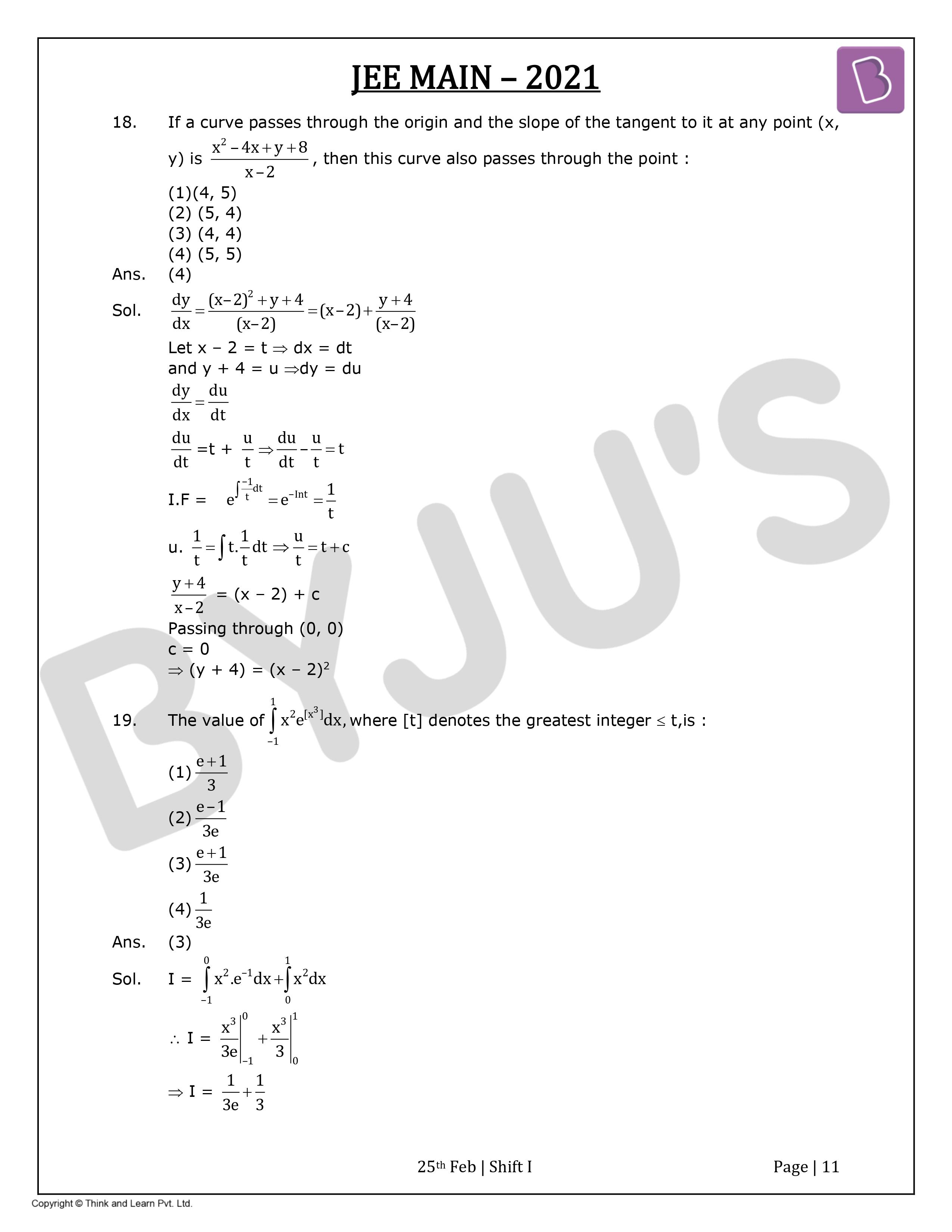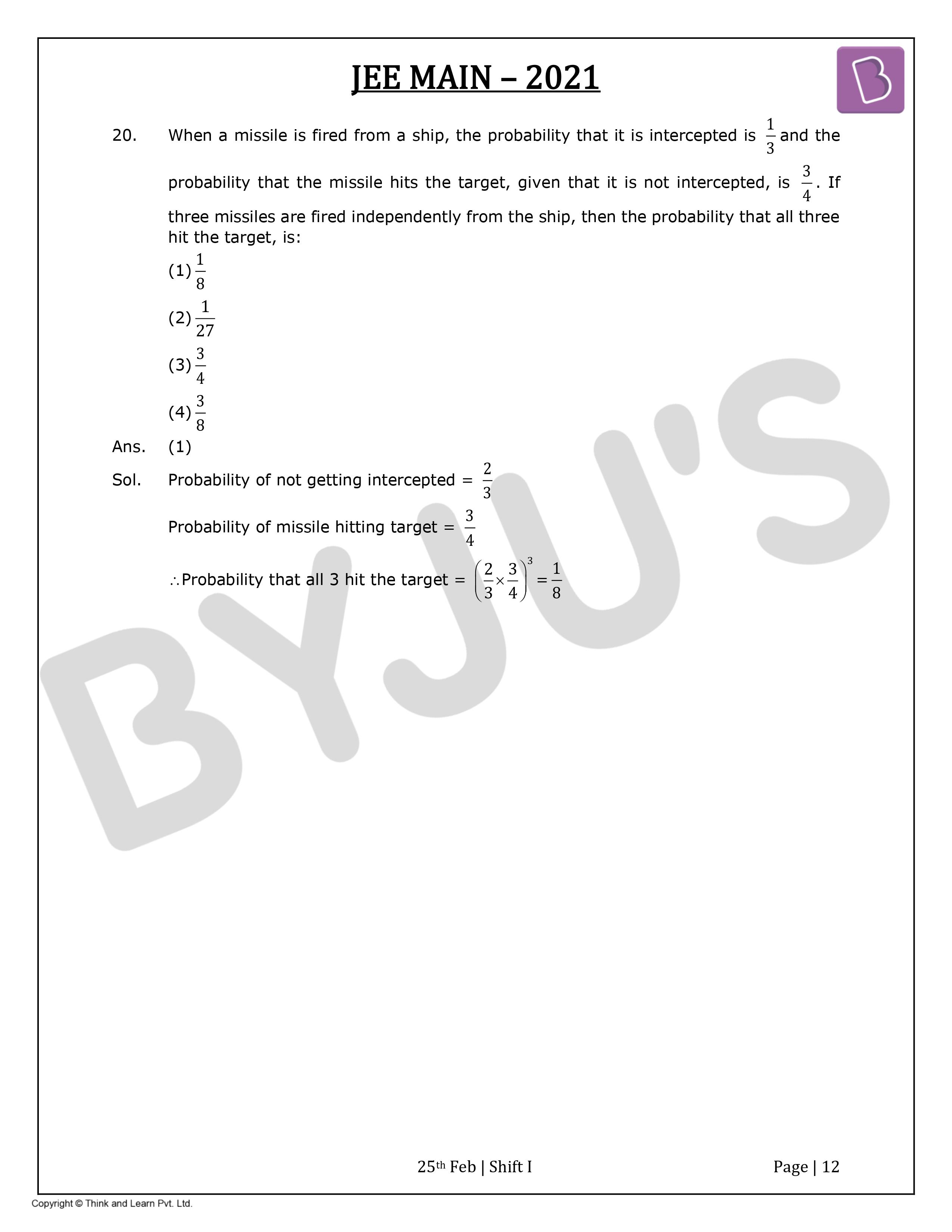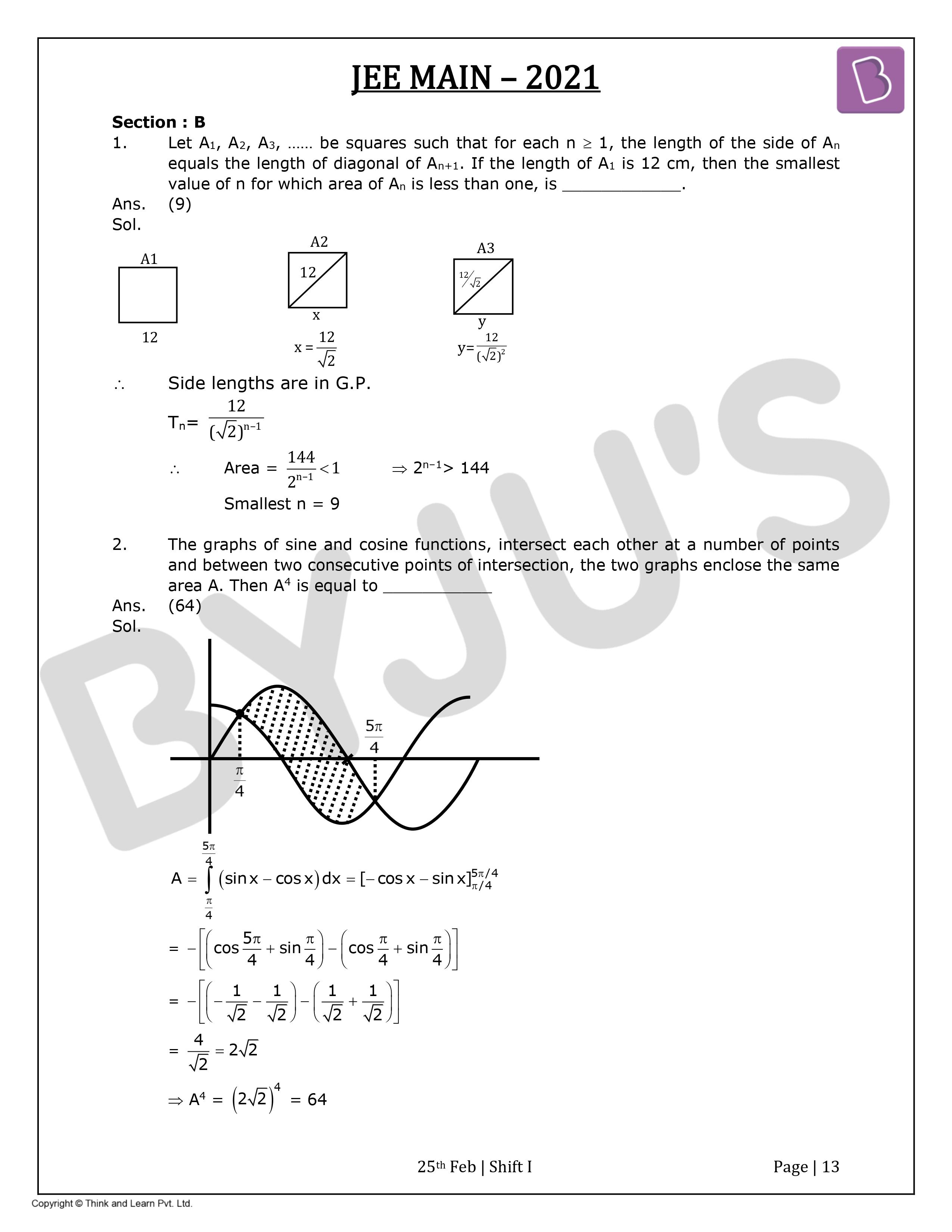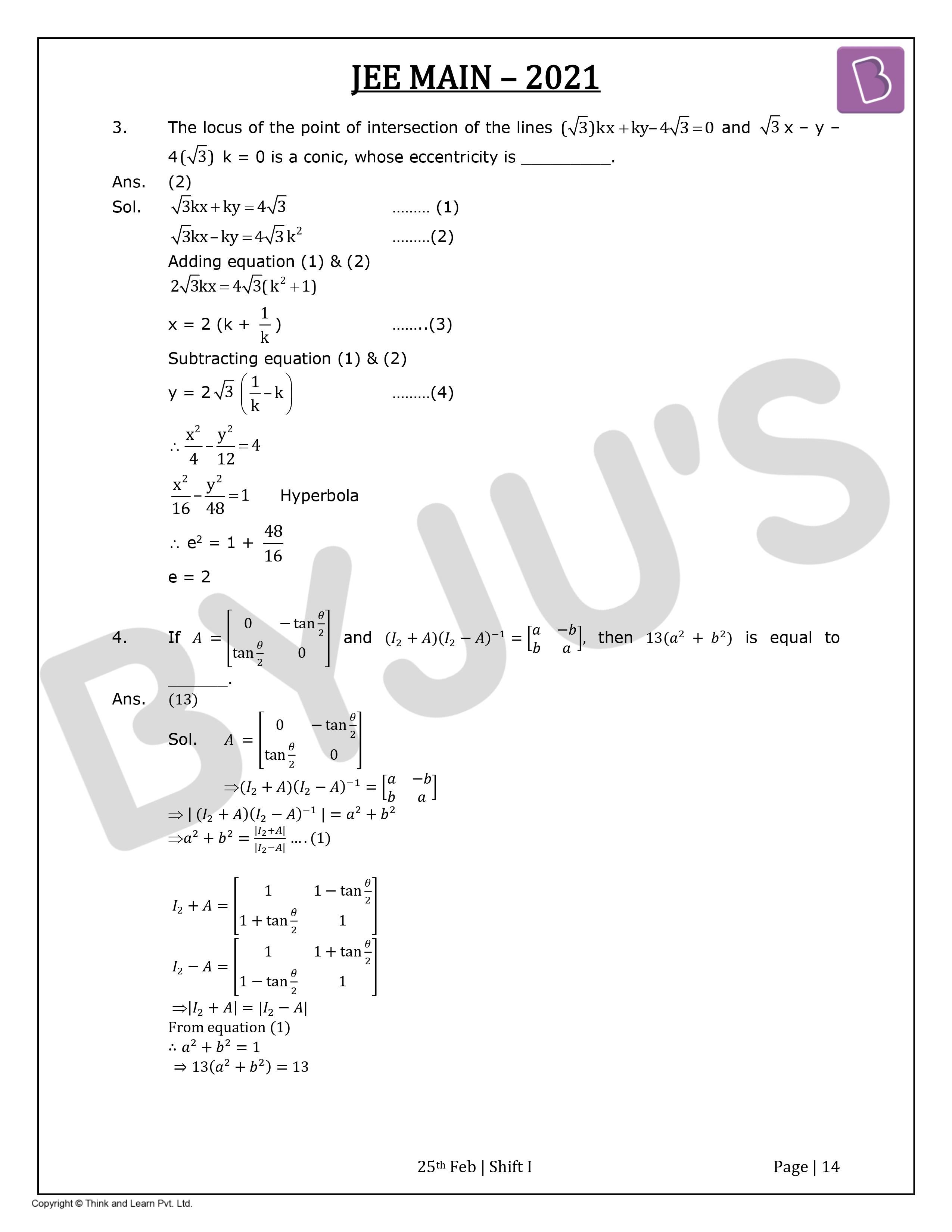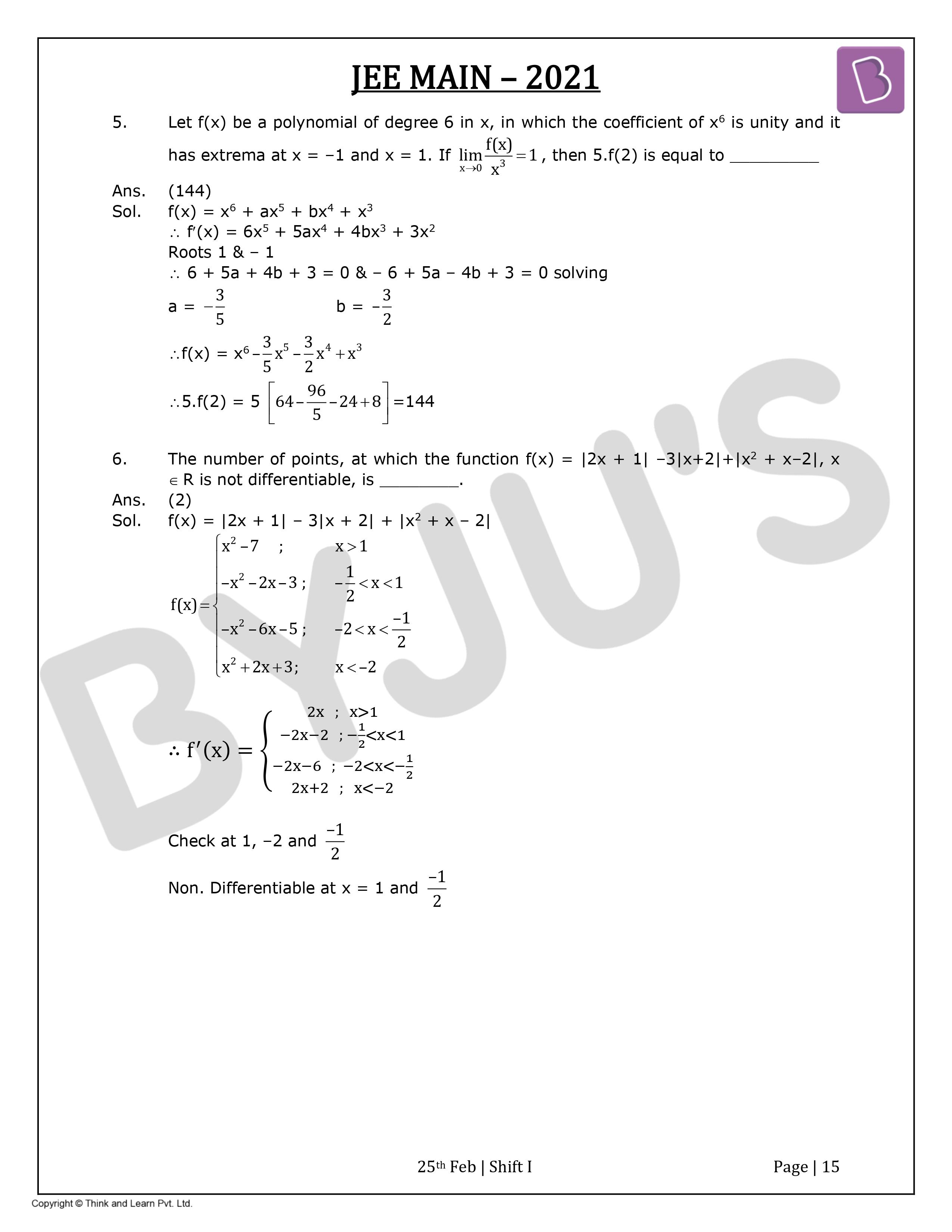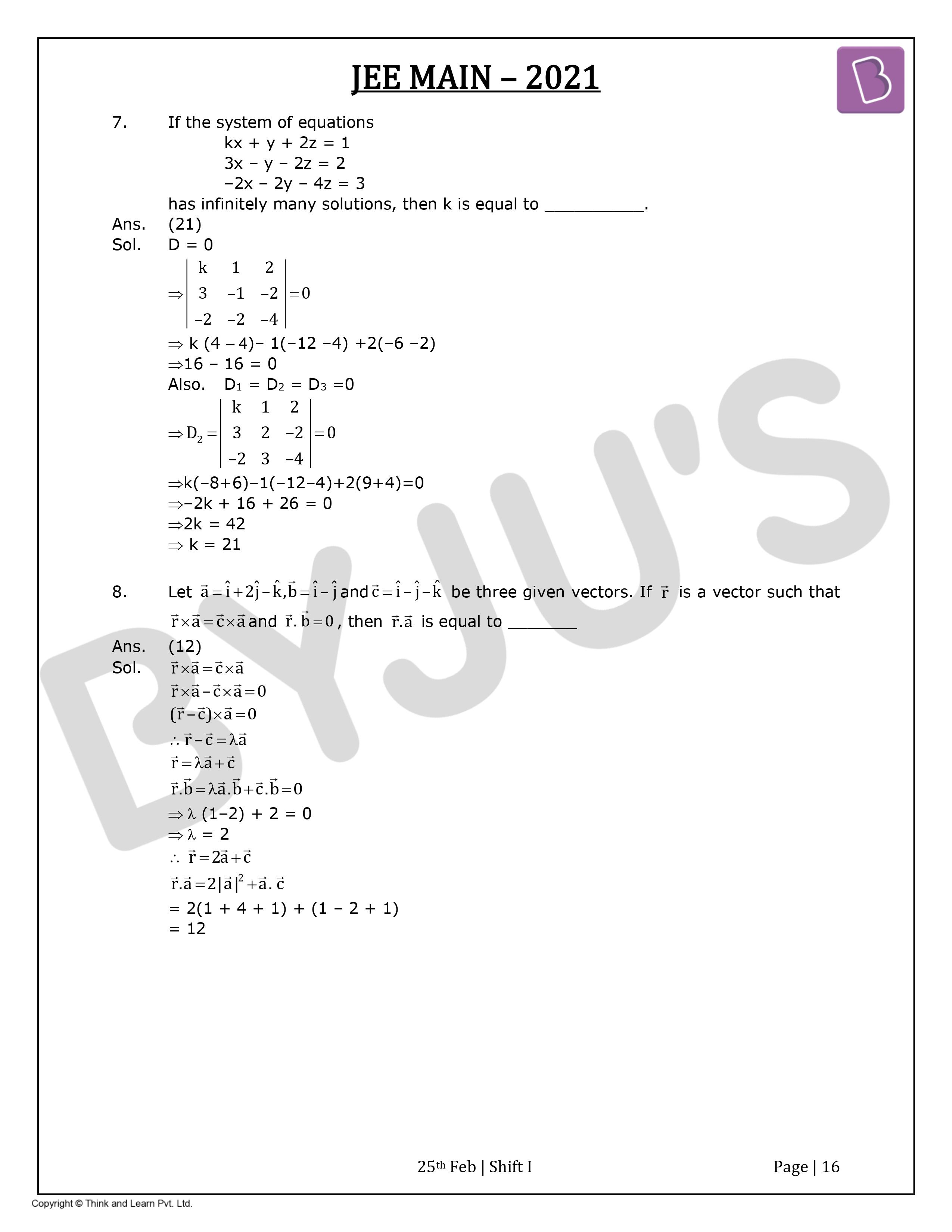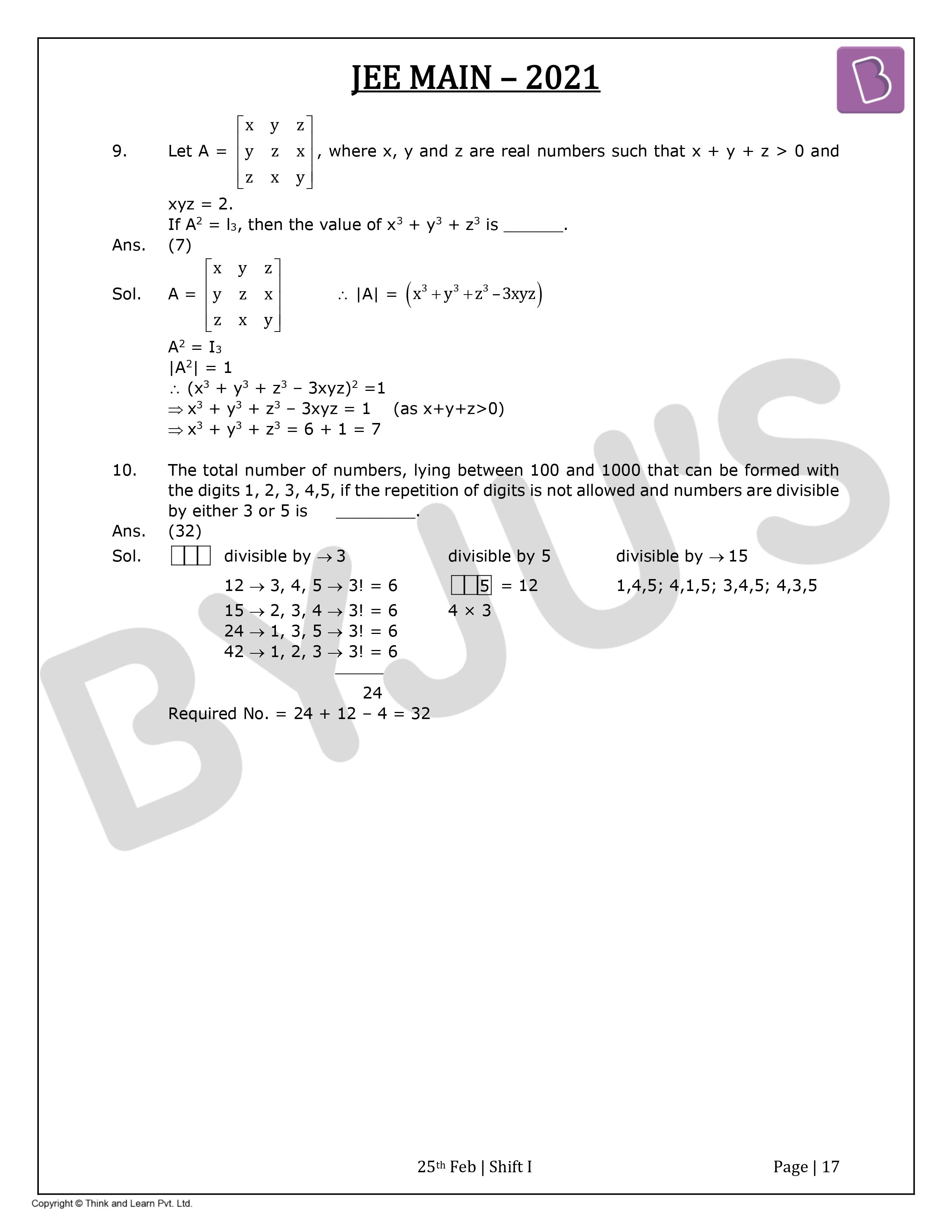Win up to 100% scholarship on Aakash BYJU'S JEE/NEET courses with ABNAT Win up to 100% scholarship on Aakash BYJU'S JEE/NEET courses with ABNAT

# JEE Main 2021 Maths Paper With Solutions Feb 25 Shift 1

Accurate solutions for each question is also provided. Students can go through these well-written solutions and learn how to formulate the correct answers. On the other hand, candidates after going through several JEE Main question papers can figure out the important topics and even predict the expected questions for the upcoming exam. In a more simple sense, students will also get a bigger picture of the overall exam pattern of JEE Main and how the paper is presented. The JEE Main 2021 Maths question paper for Feb 25 shift 1 given here will aid students to eventually be well prepared to face the upcoming exam. View and download the Maths question paper PDF below.

JEE Main 2021 25th February Shift 1 Maths Question Paper

SECTION A

Question 1: The coefficients a,b and c of the quadratic equation, ax2 + bx + c = 0 are obtained by throwing a dice three times. The probability that this equation has equal roots is :

a. 1/54
b. 1/72
c. 1/36
d. 5/216

ax2 + bx + c = 0

a,b,c ∈ {1,2,3,4,5,6}

n(s) = 6 × 6 × 6 = 216

D=0 ⇒ b2 = 4ac

ac = b2/4, If b = 2, ac = 1 ⇒ a = 1,c = 1

If b = 4, ac = 4 ⇒ a = 1, c = 4

a = 4, c = 1

a = 2, c = 2

If b = 6, ac = 9 ⇒ a = 3, c = 3

Therefore, probability = 5/216

Question 2: Let α be the angle between the lines whose direction cosines satisfy the equations l +m – n = 0 and l2 + m2 – n2 = 0. Then the value of sin4 α + cos4 α is :

a. 3/4
b. 1/2
c. 5/8
d. 3/8

Given that l+m=n ….(1)

l2 + m2 – n2 = 0 ….(2)

Squaring equation (1)

l2 + m2 + 2lm = n2 ….(3)

From equations (2) and (3)

lm=0 ⇒ l = 0 or m = 0

Case (1) : l = 0

⇒ m=n

⇒ l2 + m2 + n2 = 0

⇒ m=n = ±1/(√2 )

∴(l,m,n) = (0, 1/√2, 1/√2) or (0, -1/√2, -1/√2)

Case (2): m = 0

⇒ l = n

⇒ l2 + m2 + n2 = 0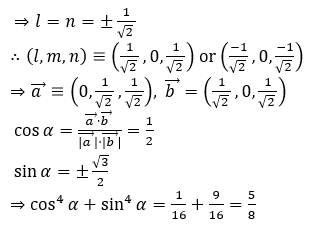Question 3: The value of the integral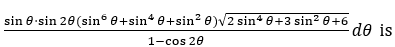(where c is a constant of integration)

a. 1/18[9 – 2 sin6 θ – 3 sin4 θ – 6 sin2 θ ](3/2) + c
b. 1/18[11 – 18 sin2 θ + 9 sin4 θ – 2 sin6 θ ](3/2) + c
c. 1/18[11 – 18 cos2 θ + 9 cos4 θ – 2 cos6 θ ](3/2) + c
d. 1/18[9 – 2 cos6 θ – 3 cos4 θ – 6 cos2 θ ](3/2) + c

Using trig identities, sin2A = 2sinAcosA and 1-cos2A = 2sin2A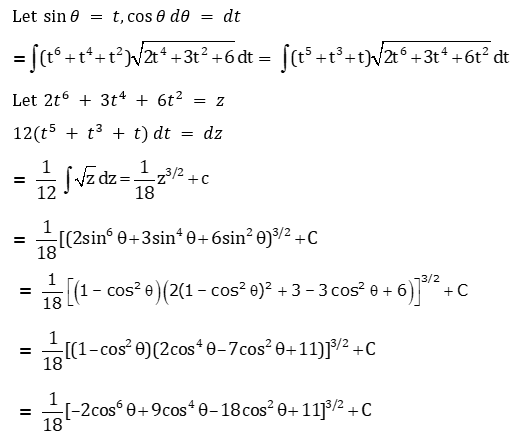Question 4: A man is observing, from the top of a tower, a boat speeding towards the tower from a certain point A, with uniform speed. At that point, the angle of depression of the boat with the man’s eye is 30° (Ignore man’s height). After sailing for 20 seconds towards the base of the tower (which is at the level of water), the boat has reached point B, where the angle of depression is 45°. Then the time taken (in seconds) by the boat from B to reach the base of the tower is :

a. 10(√3-1)
b. 10√3
c. 10
d. 10(√3+1)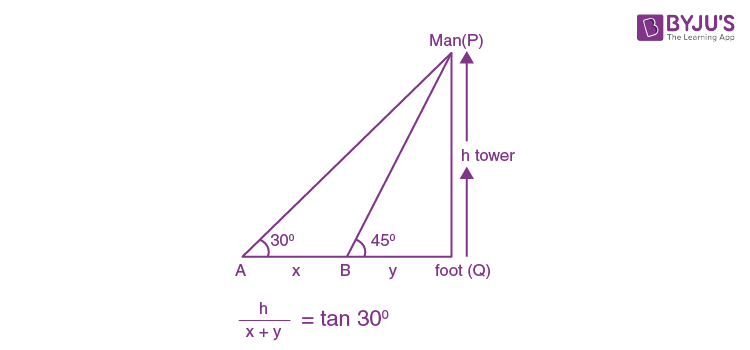x + y = √3h ….…….(1)

Also,

h/y = tan 45o

h = y …….(2)

put in (1)

x + y = √3y

x = (√3 – 1)y

x/20=v (speed)

Therefore, time taken to reach

Foot from B

= y/V

= x/(√3-1)x . 20

= 10 (√3 + 1)

Question 5: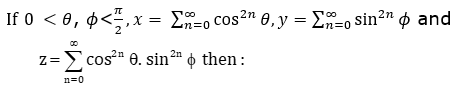a. xyz = 4
b. xy – z = (x + y)z
c. xy + yz + zx = z
d. xy + z = (x+y)z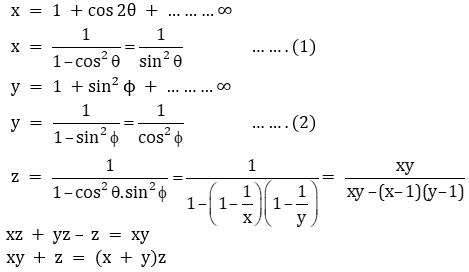Question 6: The equation of the line through the point (0,1,2) and perpendicular to the line

$$\begin{array}{l}\frac{x-1}{2} = \frac{y+1}{3} = \frac{z-1}{-2}\end{array}$$
is :

a.

$$\begin{array}{l}\frac{x}{-3} = \frac{y-1}{4} = \frac{z-2}{3}\end{array}$$

b.
$$\begin{array}{l}\frac{x}{3} = \frac{y-1}{4} = \frac{z-2}{3}\end{array}$$

c.
$$\begin{array}{l}\frac{x}{3} = \frac{y-1}{-4} = \frac{z-2}{3}\end{array}$$

d.
$$\begin{array}{l}\frac{x}{3} = \frac{y-1}{4} = \frac{z-2}{-3}\end{array}$$

$$\begin{array}{l}\frac{x-1}{2} = \frac{y+1}{3} = \frac{z-1}{-2}=\lambda\end{array}$$

Any point on this line (2λ + 1, 3λ – 1, -2λ + 1)

Direction ratio of given line

$$\begin{array}{l}\vec{d_{1}}\equiv (2, 3, -2)\end{array}$$

Direction ratio of the line to be found

$$\begin{array}{l}\vec{d_{2}}\equiv (2\lambda + 1, 3\lambda -2, -2\lambda -1)\end{array}$$

∴

$$\begin{array}{l}\vec{d_{2}}.\vec{d_{2}} = 0\end{array}$$

λ = 2 / 17

Direction ratio of line (21, -28, -21) ≡ (3, -4, -3) ≡ (-3, 4, 3)

Question 7: The statement A→ (B → A) is equivalent to:

a. A → (A ᐱ B)
b. A → (A ᐯ B)
c. A → (A → B)
d. A → (A ↔ B)

A → (B → A)

⇒ A → (∼ B ᐯ A)

⇒ ∼ A ᐯ (∼ B ᐯ A)

⇒ ∼ B ᐯ (∼ A ᐯ A)

⇒ ∼ B ᐯ t

= t (tautology)

From options:

(b) A → (A ᐯ B)

⇒ ∼ A ᐯ (A ᐯ B)

⇒ (∼ A ᐯ A) ᐯ B

⇒ t ᐯ B

⇒ t

Question 8: The integer k, for which the inequality x2 – 2(3k – 1)x + 8k2 – 7 >0 is valid for every x in R is :

a. 3
b. 2
c. 4
d. 0

D < 0

(2(3k-1))2 – 4(8k2 – 7) < 0

4(9k2 – 6k + 1) – 4(8k2 – 7) < 0

k2 – 6 k + 8 < 0

(k–4)(k–2)<0

2< k < 4

then k = 3

Question 9: A tangent is drawn to the parabola y2 = 6x which is perpendicular to the line 2x + y =1. Which of the following points does NOT lie on it?

a. (0,3)
b. (-6,0)
c. (4,5)
d. (5,4)

Equation of tangent : y = mx + 3/(2m)

mT = (1/2) (Because perpendicular to line 2x + y = 1)

Therefore, tangent is: y = x/2 + 3 ⇒ x – 2y + 6 = 0

Substitute given options in the above equation and check.

We can find that (5, 4) does not lie on the line.

Hence option (d) is the answer.

Question 10: Let f, g: N→N such that f(n + 1)= f(n)+ f(1) for all n ∈ N and g be any arbitrary function. Which of the following statements is NOT true ?

a. f is one-one
b. If fog is one-one, then g is one-one
c. If g is onto, then fog is one-one
d. If f is onto, then f(n) = n for all n ∈ N

f(n + 1) = f(n) +f(1)

⇒ f(n + 1)- f(n)= f(1) → A.P. with common difference = f(1)

General term = Tn = f(1) + (n-1)f(1) = nf(1)

⇒f(n) = nf(1)

Clearly f(n) is one-one.

For fog to be one-one, g must be one-one.

For f to be onto, f(n) should take all the values of natural numbers.

As f(x) is increasing, f(1)=1

⇒f(n)=n

If g is many one, then fog is many one. So “if g is onto, then fog is one-one” is incorrect.

Question 11: Let the lines (2 – i)z = (2 + i)

$$\begin{array}{l}\bar{z}\end{array}$$
and (2 + i)z + (i – 2)
$$\begin{array}{l}\bar{z}\end{array}$$
– 4i = 0, (here i2 = –1) be normal to a circle C. If the line iz +
$$\begin{array}{l}\bar{z}\end{array}$$
+ 1 + i = 0 is tangent to this circle C, then its radius is :

a. 3/√2
b. 3√2
c. 3/(2√2)
d. 1/(2√2)

(2–i)z = (2+i)

$$\begin{array}{l}\bar{z}\end{array}$$

⇒(2–i)(x+ iy)=(2+i)(x–iy)

⇒2x–ix + 2iy + y=2x+ ix–2iy+y

⇒2ix –4iy=0

L1 ∶ x–2y=0

⇒(2+i)z+(i –2)

$$\begin{array}{l}\bar{z}\end{array}$$
–4i=0.

⇒(2+i) (x + iy)+(i –2)(x–iy)– 4i = 0.

⇒2x +ix + 2iy – y + ix – 2x + y +2iy – 4i =0

⇒2ix + 4iy – 4i =0

L2 ∶ x+2y–2 = 0

Solve L1 and L2

4y=2 or y=1/2

∴ x = 1

Centre(1, 1/2)

L3∶ iz +

$$\begin{array}{l}\bar{z}\end{array}$$
+ 1 + i = 0

⇒i(x+iy)+x–iy+1+i=0

⇒ix–y+x–iy+1+i=0

⇒(x–y+1)+i(x–y+1)=0

Radius = distance from (1, 1/2) to x – y + 1 = 0

r = (1-1/2+1)/ √2

r = 3/2√2

Question 12: All possible values of θ ∈ [0, 2π] for which sin2θ + tan 2θ > 0 lie in

a.

$$\begin{array}{l}\left ( 0,\frac{\pi }{2} \right )\cup \left ( \pi,\frac{3\pi }{2} \right )\end{array}$$

b.
$$\begin{array}{l}\left ( 0,\frac{\pi }{4} \right )\cup \left (\frac{\pi }{2},\frac{3\pi }{4} \right )\cup \left (\pi,\frac{5\pi }{4} \right )\cup \left (\frac{3\pi }{2},\frac{7\pi }{4} \right )\end{array}$$

c.
$$\begin{array}{l}\left ( 0,\frac{\pi }{2} \right )\cup \left (\frac{\pi }{2},\frac{3\pi }{4} \right )\cup \left (\pi,\frac{7\pi }{6} \right )\end{array}$$

d.
$$\begin{array}{l}\left ( 0,\frac{\pi }{4} \right )\cup \left (\frac{\pi }{2},\frac{3\pi }{4} \right )\cup \left (\frac{3\pi }{2},\frac{11\pi }{6} \right )\end{array}$$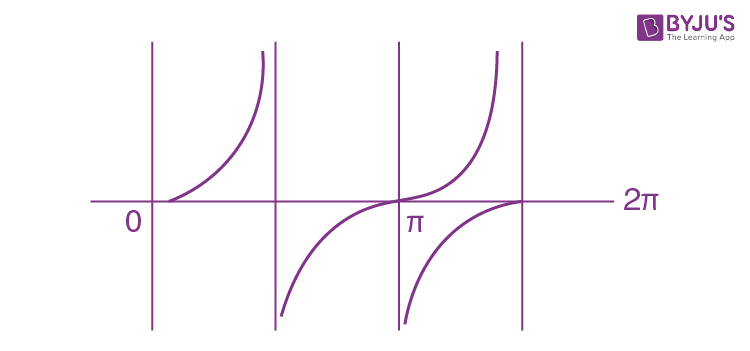tan 2θ(1+cos 2θ) >0 (since 1+cos 2θ>0)

2θ ∈ (0, π/2) ⋃(π, 3π/2) ⋃(2π, 5π/2)⋃(3π, 7π/2)

=> (0, π/4) ⋃(π/2, 3π/4) ⋃(π, 5π/4)⋃(3π/2, 7π/4)

Question 13: The image of the point (3,5) in the line x – y + 1 = 0, lies on :

a. (x – 2)2 + (y – 4) 2 =4
b. (x – 4) 2 + (y + 2)2 =16
c. (x – 4)2 + (y – 4)2 = 8
d. (x – 2)2 + (y – 2)2 =12

Image of P(3, 5) on the line x – y + 1 = 0 is

$$\begin{array}{l}\frac{x-3}{1} = \frac{y-5}{-1} = \frac{-2(3-5+1)}{2} = 1\end{array}$$

x = 4, y = 4

Image is (4, 4)

Which lies on (x – 2)2 + (y – 4)2 = 4

Question 14: If Rolle’s theorem holds for the function f(x) = x3 – ax2 + bx – 4, x ∈ [1, 2] with f'(4/3) = 0, then ordered pair (a, b) is equal to :

a. (–5, 8)
b. (5, 8)
c. (5, –8)
d. (–5, –8)

f(1) = f(2)

⇒ 1 – a + b –4 = 8 – 4a + 2b –4

3a – b = 7 …(1)

f’(x) = 3x2 – 2ax + b

⇒f’(4/3) = 0 ⇒ 3 x 16/9 – 8a/3 + b =0

⇒–8a + 3b = –16 …(2)

a = 5, b = 8

Question 15: If the curves,

$$\begin{array}{l}\frac{x^2}{a}+\frac{y^2}{b}\end{array}$$
and
$$\begin{array}{l}\frac{x^2}{c}+\frac{y^2}{d}\end{array}$$
intersect each other at an angle of 90°, then which of the following relations is true ?

a. a + b = c + d
b. a- b = c – d
c. ab = (c+d)/(a+b)
d. a-c = b+d

$$\begin{array}{l}\frac{x^2}{a}+\frac{y^2}{b}\end{array}$$
………(1)

diff :

$$\begin{array}{l}\frac{2x}{a}+\frac{2y}{b}\frac{dy}{dx} = 0\end{array}$$
$$\begin{array}{l}\frac{y}{b}\frac{dy}{dx} = \frac{-x}{a}\end{array}$$
$$\begin{array}{l}\frac{dy}{dx} = \frac{-bx}{ay}\end{array}$$
……(2)

$$\begin{array}{l}\frac{x^2}{c}+\frac{y^2}{d}\end{array}$$
………(3)

Diff :

$$\begin{array}{l}\frac{dy}{dx} = \frac{-dx}{cy}\end{array}$$
………(4)

m1m2 = –1 ⇒

$$\begin{array}{l}\frac{-bx}{ay} \times \frac{-dx}{cy} = -1\end{array}$$

⇒ bdx2 = – acy2…….(5)

(1)–(3) ⇒ (1/a – 1/c)x2 + (1/b – 1/d) y2 = 0

Solve above equation using 5

⇒ (c – a) – (d – b) = 0

⇒ c – a = d – b

⇒ c – d = a – b

Question 16: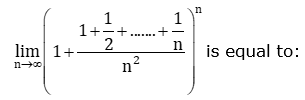a. 1/2
b. 1/e
c. 1
d. 0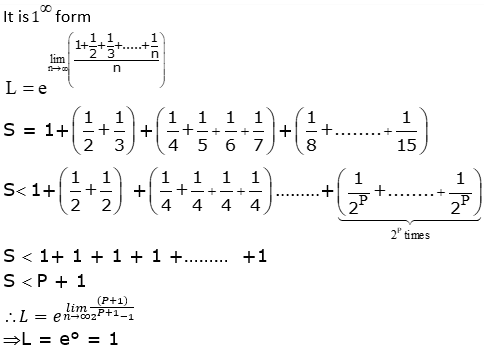Question 17: The total number of positive integral solutions (x, y, z) such that xyz = 24 is

a. 36
b. 45
c. 24
d. 30

x.y.z = 24

x.y.z=23.31

x=2(a_1 )⋅3(b_1 )

y = 2(a_2 )⋅3(b_2 )

z = 2(a_3 )⋅3(b_3 )

a1, a2, a3 ∈ {0,1,2,3}

b1, b2, b3 ∈ {0,1}

Case 1: a1 + a2 + a3 =3

Non negative solution = (3+3-1) C(3-1) = 5C2 = 10

Case 2: b1 + b2 + b3 = 1

Non negative solution = (1+3-1) C(3-1) = 3C2 = 3

∴ Total solutions =10×3=30

Question 18: If a curve passes through the origin and the slope of the tangent to it at any point (x, y) is

$$\begin{array}{l}\frac{x^2-4x+y+8}{x-2}\end{array}$$
, then this curve also passes through the point :

a. (4, 5)
b. (5, 4)
c. (4, 4)
d. (5, 5)

$$\begin{array}{l}\frac{dy}{dx} = \frac{(x-2)^2 +y+4}{x-2} = (x-2) + \frac{y+4}{x-2}\end{array}$$

Let x – 2 = t ⇒ dx = dt

and y + 4 = u ⇒dy = du

dy/dx = du/dt

du/dt = t + u/t ⇒ du/dt – u/t = t

Here, IF = 1/t

u. (1/t) = ∫ t.(1\t) dt

⇒ u/t = t + c

⇒ (y+4)/(y-2) = (x – 2) + c

Passing through (0, 0)

c = 0

⇒ (y + 4) = (x – 2)2

Question 19: The value of

$$\begin{array}{l}\int_{-1}^1 x^2 e^{x^3}dx\end{array}$$
where [t] denotes the greatest integer ≤ t,is :

a. (e+1)/3
b. (e-1)/3e
c. (e+1)/3e
d. 1/3e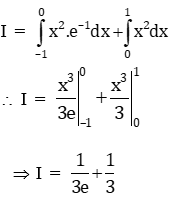Question 20: When a missile is fired from a ship, the probability that it is intercepted is 1/3 and the probability that the missile hits the target, given that it is not intercepted, is 3/4. If three missiles are fired independently from the ship, then the probability that all three hit the target, is:

a. 1/8
b. 1/27
c. 3/4
d. 3/8

Probability of not getting intercepted = 2/3

Probability of missile hitting target = 3/4

Probability that all 3 hit the target = (2/3 x3/4)3 = 1/8

Section B

Question 1: Let A1, A2, A3,…. be squares such that for each n ≥ 1, the length of the side of An equals the length of diagonal of An+1. If the length of A1 is 12 cm, then the smallest value of n for which area of An is less than one is ____________.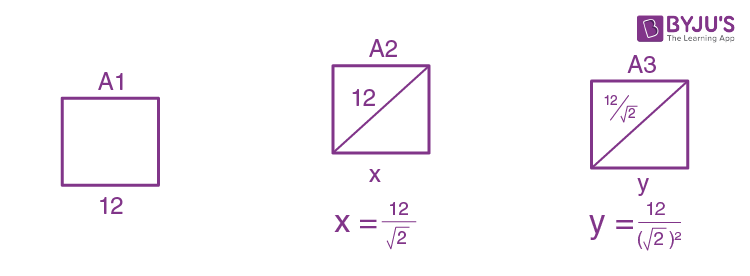Side lengths are in GP.

Tn = 12/(√2)n-1

Area = 144/2n-1 <1

=> 2n-1 > 144

Smallest n = 9

Question 2: The graphs of sine and cosine functions, intersect each other at a number of points and between two consecutive points of intersection, the two graphs enclose the same area A. Then A4 is equal to ___________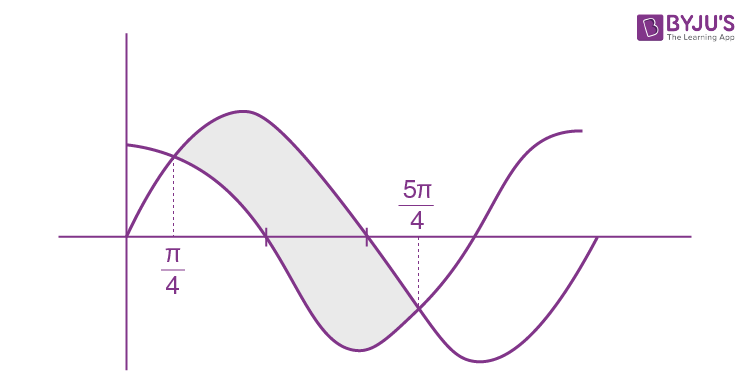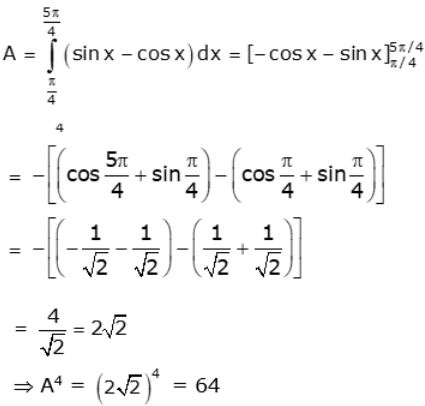Question 3: The locus of the point of intersection of the lines (√3)kx + ky – 4√3 = 0 and √3x – y – 4√3 k = 0 is a conic, whose eccentricity is _________.

(√3)kx + ky – 4√3 = 0 …(1)

√3kx -ky= 4√3 k2 …(2)

2√3 kx = 4√3(k^2 + 1)

x = 2 (k + 1/k) ….(3)

Subtracting equation (1) & (2)

y = 2√3(1/k – k) ………(4)

x2/4 – y2/12 = 4

x2/16 – y2/48 = 1 – Hyperbola

e2 = 1 + 48/16

or e = 2

Question 4: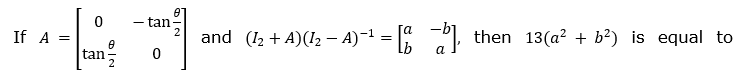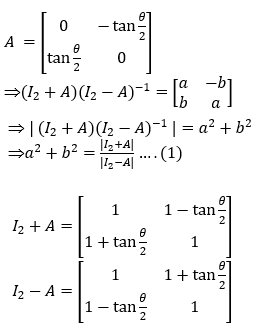|I2 + A|=|I2 – A|

From equation (1)

∴a2 + b2 = 1

⇒13(a2 + b2) = 13

Question 5: Let f(x) be a polynomial of degree 6 in x, in which the coefficient of x6 is unity and it has extrema at x = –1 and x = 1. If

$$\begin{array}{l}\lim_{x \to 0}\frac{f(x)}{x^3}=1\end{array}$$
, then 5.f(2) is equal to _________.

f(x) = x6 + ax5 + bx4 + x3

f’(x) = 6x5 + 5ax4 + 4bx3 + 3x2

Roots 1 & – 1

Therefore, 6 + 5a + 4b + 3 = 0 & – 6 + 5a – 4b + 3 = 0 solving

a = -3/5 and b = -3/2

f(x) = x6 – (3/5)x5 – (3/2)x4 + x3

5.f(2) = 5 [64 – 96/5 – 24 + 8] = 144

Question 6: The number of points, at which the function f(x) = |2x + 1| – 3|x+2|+|x2 + x–2|, x ∈ R is not differentiable, is ________.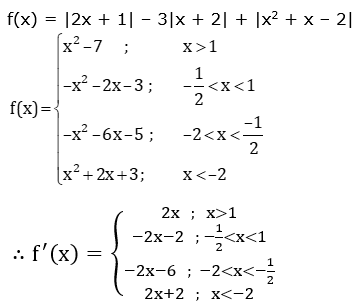Check at 1, –2 and -1/2

Non-Differentiable at x = 1 and -1/2

Question 7: If the system of equations

kx + y + 2z = 1

3x – y – 2z = 2

–2x – 2y – 4z = 3

has infinitely many solutions, then k is equal to __________.

D = D1 = D2 = D3 = 0

Choose D2 = 0

k(–8+6)–1(–12–4)+2(9+4)=0

–2k + 16 + 26 = 0

2k = 42

k = 21

Question 8: Let

$$\begin{array}{l}\vec{a} = \hat{i} + 2 \hat{j} – \hat{k}, \vec{b} = \hat{i} – \hat{j}\end{array}$$
and
$$\begin{array}{l}\vec{c} = \hat{i} – \hat{j} – \hat{k}\end{array}$$
be three given vectors. If
$$\begin{array}{l}\vec{r}\end{array}$$
is a vector such that
$$\begin{array}{l}\vec{r} \times \vec{a} = \vec{c} \times \vec{a}\end{array}$$
and
$$\begin{array}{l}\vec{r} . \vec{b} = 0\end{array}$$
, then
$$\begin{array}{l}\vec{r} . \vec{a} = 0\end{array}$$
is equal to _______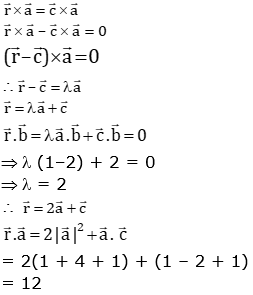Question 9: Let A =

$$\begin{array}{l}\begin{bmatrix} x &y &z \\ y &z &x \\ z & x &y \end{bmatrix}\end{array}$$
, where x, y and z are real numbers such that x + y + z > 0 and xyz = 2. If A2 = l3, then the value of x3 + y3 + z3 is ______.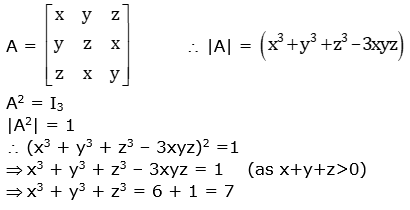Question 10: The total number of numbers, lying between 100 and 1000 that can be formed with the digits 1, 2, 3, 4,5, if the repetition of digits is not allowed and numbers are divisible by either 3 or 5 is ________.